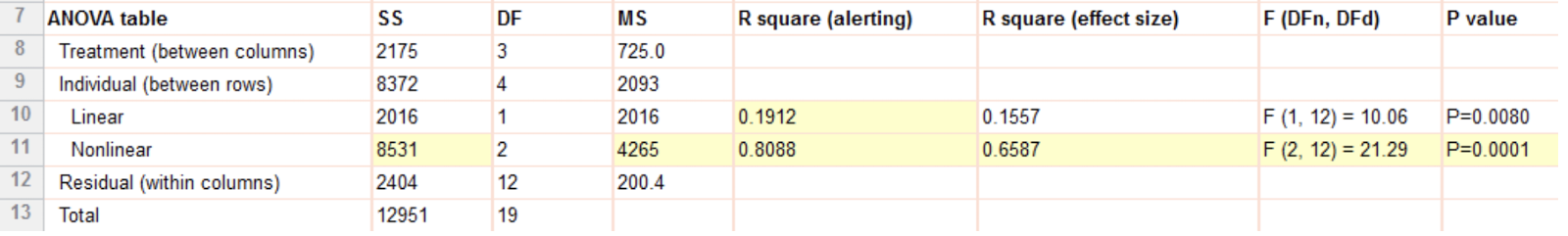KNOWLEDGEBASE - ARTICLE #2108

# Fixed bug in test for linear trend after repeated measures one-way ANOVA

One of the followup tests after repeated measures one-way ANOVA is the test for linear trend.

We recently discovered a bug in some of the associated results. This bug, fixed in Prism 7.05 Windows and 7.0e Mac, resulted in incorrect results for the values highlighted below. R square (alerting) and P value (nonlinear) were too low. The SS(nonlinear), MS(nonlinear), F(nonlinear), nonlinear R square (alerting) and nonlinear R square (effect size) were too high.Note that the actual test for linear trend (with the exception of the alerting R2) has been correct. It is the test for nonlinear trend that was incorrect. Also note that the bug is only after repeated measures one-way ANOVA, not the test for trend following ordinary one-way ANOVA.

Math details.

1) Summary for nonlinear components

SS(nonlinear) was calculated as follows (that is not correct):
SS(nonlinear) = SS(treatment) - SS(linear) + SS(subject)

The correct expression is:
SS(nonlinear) = SS(treatment) - SS(linear)

As a result, MS, R^2, F, DF(nonlinear) and P value were incorrect.

Correct expression that can be used for obtaining correct results (by hand):

SS(nonlinear) = SS(treatment) - SS(linear)
MS(nonlinear) = SS(nonlinear) / DF(nonlinear)
R square (alerting) = SS(nonlinear)/ SS(treatment)
R square (effect size) = SS(nonlinear) / SS(total)
F(nonlinear) = MS(nonlinear) / MS(residuals)
p value = PFromF(F(nonlinear), DF(nonlinear), DF(residuals))

2) R square (altering) for linear component

Incorrect expression:
R square (alerting) = SS(linear)/ (SS(treatment) + SS(subjects))

Correct expression:
R square (alerting) = SS(linear)/ SS(treatment)

## Explore the Knowledgebase

Analyze, graph and present your scientific work easily with GraphPad Prism. No coding required.